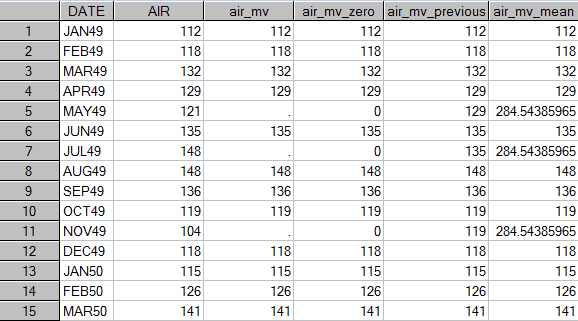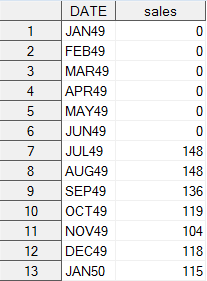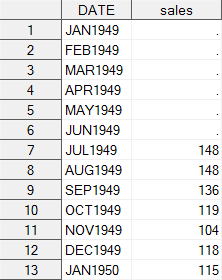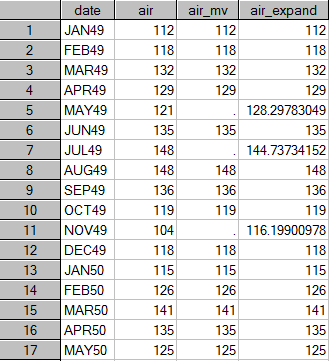## Replace MISSING VALUES in TIMESERIES DATA using PROC EXPAND and PROC TIMESERIES

Started ‎02-04-2021 by
Modified ‎02-12-2021 by
Views 6,961

This article illustrates how you can use the EXPAND and the TIMESERIES procedure to replace missing values in timeseries data. A separate SAS Communities article "Using the TIMESERIES procedure to check the continuity of your timeseries data" focuses on the problem of missing records in your analysis data.

Note that in order to run PROC TIMESERIES and PROC EXPAND you need SAS/ETS.

# Replacing Missing Values with PROC TIMESERIES

This section discusses using the TIMESERIES procedure to replace missing values in time series data. Missing values in this context mean that the missing values occur explicitly in time series data where the value for a certain time period is missing.

PROC TIMESERIES allows you to replace missing values by using one of the replacement methods listed in the table below. These methods are controlled with the option SETMISS. For details, refer to the documentation of PROC TIMESERIES, section ID statement, SETMISS option.

 Option value Missing values are set to Any number. (for example, 0 to replace missing values with zero) MISSING Missing AVERAGE Average value of the time series MEDIAN Median value of the time series MINIMUM Minimum value of the time series MAXIMUM Maximum value of the time series FIRST First non-missing value LAST Last non-missing value PREVIOUS Previous non-missing value NEXT Next non-missing value

# PROC TIMESERIES Examples

In order to demonstrate how to use PROC TIMESERIES to impute missing values, artificial missing values are inserted in the SASHELP.AIR data set. A new variable, AIR_MV, is created as a copy of the AIR variable and approximately 15% of the missing values are inserted:

``````data air_missing;
set sashelp.air;
if uniform(12345) < 0.15 then air_mv = .;
else air_mv = air;
run;``````

The following table shows the first 17 rows of table AIR_MISSING with missing values for April and July 1949 and March 1950. Note that the AIR variable is only kept for explanatory purposes. In a real-world situation, variable AIR would not exist, and the missing values found in AIR_MV would need to be replaced for the analysis.PROC TIMESERIES can now be used to set missing values to zero with the following code:

``````proc timeseries data = air_missing
out = air_setmissing_zero;
id date interval =month setmiss=0;
var air_MV;
run;``````

Another example shows how to replace missing values with the previous value in the time series:

`````` proc timeseries data = air_missing
out = air_setmissing_previous;
id date interval =month setmiss=PREVIOUS;
var air_MV;
run;``````

A third example shows how to replace missing values with the mean value in the time series:

``````proc timeseries data = air_missing
out = air_setmissing_mean;
id date interval =month setmiss=MEAN;
var air_MV;
run;``````

In the following table the output data sets of these three examples have been joined together for illustrative purposes.Different replacement methods in PROC TIMESERIES

In the table above only a subset of the observations of a timeseries with an upward trend are shown. As the mean for the replacement in the last column has be calculated based on all observations of the time series, it looks like as it would be to high for this subset of observations.

Note that you can also specify a BY statement with PROC TIMESERIES in order to replace missing values by BY group.

# Changing zero values to missing values

PROC TIMESERIES also offers the ability to change zero values to missing values. This is important if the data show zero values, which, for the analysis, however, should be interpreted as missing values (for example, the introduction of new products or the retirement of products in the retail or fashion industry). Here, the zero value represents different phases of the product lifecycle rather than a comparable quantity.

``````proc timeseries data=sales_original out=sales_corrected;
id date interval=month zeromiss=both;
var sales;
run;
``````

The first table shows the data before PROC TIMESERIES is applied, and second table shows the data after PROC TIMESERIES has set the leading and trailing zeros to missing. Note that beside ZEROMISS=BOTH, which replaces both leading and trailing zeros, LEFT and RIGHT can also be used as option values.Data with leading zerosData with zeros replaced by missing values

# Interpolating Missing Values in Time Series Data with PROC EXPAND

The EXPAND procedure allows you to

• convert time series data from one sampling interval to another and to interpolate missing values.
• Time series data can be collapsed from a higher frequency interval to a lower frequency interval
• or expanded from a lower frequency interval to a higher frequency interval.
• Monthly data can be aggregated into annual data, and quarterly data can be interpolated from an annual series.

Aperiodic time series that are observed at non-regular points in time can be converted into periodic estimates. For example, events that are documented at random time points can be interpolated to form weekly average event rates.

This section focuses on interpolating missing values with PROC EXPAND.

## Statistical methods in PROC EXPAND

By default, PROC EXPAND fits cubic spline curves to the non-missing values of the input variable to form continuous approximations of the time series. The respective output series are then generated from the spline approximations. Different from commonly used natural spline methods, which use zero second-derivate endpoint constraints, the SPLINE method in PROC EXPAND uses the not-a-knot method by default, which has proven to be more appropriate for time series data. For more details see the SAS documentation for PROC EXPAND, chapter “Conversion Methods”

Other methods to interpolate missing values with PROC EXPAND include:

• the JOIN method, where interpolations are calculated based on the successive straight line collection between the non-missing points.
• the STEP method, which is a discontinuous piecewise constant curve where the value is interpolated from the most recent non-missing value.

## Examples for PROC EXPAND

This example demonstrates how to use PROC EXPAND based on the data that have been used in the PROC TIMESERIES example above.

With the following SAS statements, a new variable, AIR_EXPAND, is produced that contains the non-missing values of AIR_MV as well as interpolated values for those months where AIR_MV is missing:

``````proc expand data = air_missing out = air_expand;
convert air_mv=air_expand;
id date;
run;``````

The following table shows the first 17 rows of data set AIR_EXPAND. The interpolated values are stored in variable AIR_EXPAND.Imputed values with PROC EXPAND

PROC EXPAND can also be used with a BY statement if the input data contain different time series in a cross-sectional format that are identified by one or more BY variables.

Assuming that the SASHELP.AIR data set contains a COUNTRY variable that separates the number of airline passengers by country, the previous code example would appear as follows:

``````proc expand data = air_missing out = air_expand;
convert air_mv=air_expand;
id date;
by country;
run;
``````

This example has been taken from my SAS Press book "Data Quality for Analytics Using SAS".

Further books of the author in SAS Press:

Related SAS-Communities articlesVersion history
Last update:
‎02-12-2021 08:10 AM
Updated by:Contributors
Article Labels
Article Tags